# Complementary and supplementary angles | Types of Angle Pairs | geometry

In this section we discuss about different types of angles pairs  like Complementary Angles, Supplementary Angles, Conjugate Angles & Congruent angles with examples

### Angle Pairs Definition and Examples  | Conjugate and Congruent Angles

For Basic concepts of angles and Different Types of Angles in Geometry like Zero Angle, Acute Angle, Right Angle, Obtuse angle, Straight Angle, Reflex Angle & Complete angle go through the below link

### Complementary Angles

If the sum of two angles are 90o then the angles are said to be Complementary angles. Each one of these angles is called the Complementary of the other.

Example: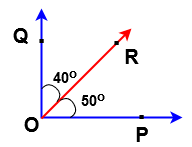From the above example  ∠POR = 50o , ∠ROQ = 40 are complementary angles

Since sum of the these two angles are 90o

i.e ∠POR + ∠ROQ  = 50o + 40o = 90o

Here ∠POR is said to be complementary angle of ∠ROQ and ∠ROQ is said to be complementary angle of ∠POR.

How to find complementary angles

If any angle of ‘ y ‘ is less than 90o then

Complementary angle of  y  = 90o  – yo

### Supplementary angles

If the sum of two angles are 180o then the angles are said to be supplementary angles,  . Each one of these angles is called the supplementary of the other.

Example: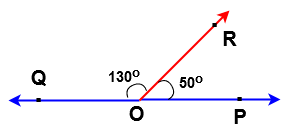From the above example  ∠POR = 50o , ∠ROQ = 130 are supplementary angles

Since sum of the these two angles are 180o

i.e ∠POR + ∠ROQ  = 50o + 130o = 180o

Here ∠POR is said to be supplementary angle of ∠ROQ and ∠ROQ is said to be supplementary angle of ∠POR.

How to find supplementary angles

If any angle of Y is less than 180o then

Supplementary angle of  y  = 180o  – yo

### Conjugate Angles:

If the sum of two angles are 360 then the angles are said to be Conjugate angles,  . Each one of these angles is called the conjugate of the other.

Example: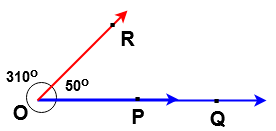From the above example  ∠POR = 50o , ∠ROQ = 310 are conjugate angles

Since sum of the these two angles are 360o

i.e ∠POR + ∠ROQ  = 50o + 310o = 310o

Here ∠POR is said to be conjugate angle of ∠ROQ and ∠ROQ is said to be conjugate angle of ∠POR.

How to find conjugate angles

If any angle of  ‘y ‘ is less than 360o then

Conjugate angle of y  = 360o  – yo

### Congruent angles:

Two angles having the same measure are known as congruent angle.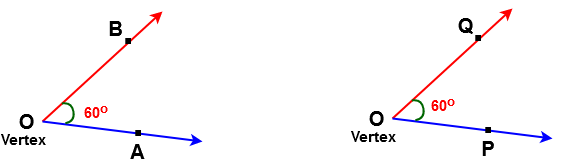In the above figure ∠AOB & ∠POQ  are congruent angles.

Since ∠AOB  =  ∠POQ  = 60o

### Angular bisector:

A ray which divides an angle into two congruent angles is called angular bisector.

Example: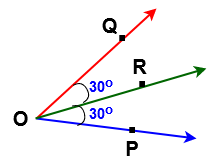In the above figure ray OR is called angular bisector of ∠POQ.

Since ∠POR = ∠ROQ = 30o